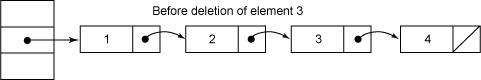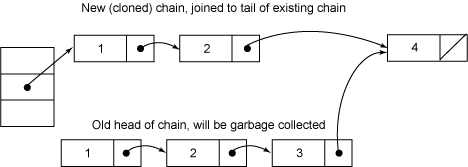# ConcurrentHashMap之实现细节

ConcurrentHashMap是Java 5中支持高并发、高吞吐量的线程安全HashMap实现。闲话少说，通过仔细阅读源代码，现在总算理解ConcurrentHashMap实现机制了，其实现之精巧，令人叹服，与大家共享之。

## 锁分离 (Lock Stripping)

ConcurrentHashMap允许多个修改操作并发进行，其关键在于使用了锁分离技术。它使用了多个锁来控制对hash表的不同部分进行的修改。ConcurrentHashMap内部使用段(Segment)来表示这些不同的部分，每个段其实就是一个小的hash table，它们有自己的锁。只要多个修改操作发生在不同的段上，它们就可以并发进行。

   /**
* The segments, each of which is a specialized hash table
*/
final Segment<K,V>[] segments;


## 不变(Immutable)和易变(Volatile)

ConcurrentHashMap完全允许多个读操作并发进行，读操作并不需要加锁。如果使用传统的技术，如HashMap中的实现，如果允许可以在hash链的中间添加或删除元素，读操作不加锁将得到不一致的数据。ConcurrentHashMap实现技术是保证HashEntry几乎是不可变的。HashEntry代表每个hash链中的一个节点，其结构如下所示：

    static final class HashEntry<K,V> {
final K key;
final int hash;
volatile V value;
final HashEntry<K,V> next;
}


## 其它

    private static int hash(int h) {
// Spread bits to regularize both segment and index locations,
// using variant of single-word Wang/Jenkins hash.
h += (h <<  15) ^ 0xffffcd7d;
h ^= (h >>> 10);
h += (h <<   3);
h ^= (h >>>  6);
h += (h <<   2) + (h << 14);
return h ^ (h >>> 16);
}


   final Segment<K,V> segmentFor(int hash) {
return segments[(hash >>> segmentShift) & segmentMask];
}


## 数据结构

public class ConcurrentHashMap<K, V> extends AbstractMap<K, V>
implements ConcurrentMap<K, V>, Serializable {
/**
* Mask value for indexing into segments. The upper bits of a
* key's hash code are used to choose the segment.
*/

/**
* Shift value for indexing within segments.
*/
final int segmentShift;

/**
* The segments, each of which is a specialized hash table
*/
final Segment<K,V>[] segments;
}


    static final class Segment<K,V> extends ReentrantLock implements Serializable {
private static final long serialVersionUID = 2249069246763182397L;
/**
* The number of elements in this segment's region.
*/
transient volatile int count;

/**
* Number of updates that alter the size of the table. This is
* used during bulk-read methods to make sure they see a
* consistent snapshot: If modCounts change during a traversal
* of segments computing size or checking containsValue, then
* we might have an inconsistent view of state so (usually)
* must retry.
*/
transient int modCount;

/**
* The table is rehashed when its size exceeds this threshold.
* (The value of this field is always <tt>(int)(capacity *
*/
transient int threshold;

/**
* The per-segment table.
*/
transient volatile HashEntry<K,V>[] table;

/**
* The load factor for the hash table.  Even though this value
* is same for all segments, it is replicated to avoid needing
* @serial
*/
}


## 实现细节

    public V remove(Object key) {
int hash = hash(key.hashCode());
return segmentFor(hash).remove(key, hash, null);
}


    V remove(Object key, int hash, Object value) {
lock();
try {
int c = count - 1;
HashEntry<K,V>[] tab = table;
int index = hash & (tab.length - 1);
HashEntry<K,V> first = tab[index];
HashEntry<K,V> e = first;
while (e != null && (e.hash != hash || !key.equals(e.key)))
e = e.next;

V oldValue = null;
if (e != null) {
V v = e.value;
if (value == null || value.equals(v)) {
oldValue = v;
// All entries following removed node can stay
// in list, but all preceding ones need to be
// cloned.
++modCount;
HashEntry<K,V> newFirst = e.next;
for (HashEntry<K,V> p = first; p != e; p = p.next)
newFirst = new HashEntry<K,V>(p.key, p.hash,
newFirst, p.value);
tab[index] = newFirst;
count = c; // write-volatile
}
}
return oldValue;
} finally {
unlock();
}
}V put(K key, int hash, V value, boolean onlyIfAbsent) {
lock();
try {
int c = count;
if (c++ > threshold) // ensure capacity
rehash();
HashEntry<K,V>[] tab = table;
int index = hash & (tab.length - 1);
HashEntry<K,V> first = tab[index];
HashEntry<K,V> e = first;
while (e != null && (e.hash != hash || !key.equals(e.key)))
e = e.next;

V oldValue;
if (e != null) {
oldValue = e.value;
if (!onlyIfAbsent)
e.value = value;
}
else {
oldValue = null;
++modCount;
tab[index] = new HashEntry<K,V>(key, hash, first, value);
count = c; // write-volatile
}
return oldValue;
} finally {
unlock();
}
}


     V get(Object key, int hash) {
if (count != 0) { // read-volatile
HashEntry<K,V> e = getFirst(hash);
while (e != null) {
if (e.hash == hash && key.equals(e.key)) {
V v = e.value;
if (v != null)
return v;
}
e = e.next;
}
}
return null;
}


get操作不需要锁。第一步是访问count变量，这是一个volatile变量，由于所有的修改操作在进行结构修改时都会在最后一步写count变量，通过这种机制保证get操作能够得到几乎最新的结构更新。对于非结构更新，也就是结点值的改变，由于HashEntry的value变量是volatile的，也能保证读取到最新的值。接下来就是对hash链进行遍历找到要获取的结点，如果没有找到，直接访回null。对hash链进行遍历不需要加锁的原因在于链指针next是final的。但是头指针却不是final的，这是通过getFirst(hash)方法返回，也就是存在table数组中的值。这使得getFirst(hash)可能返回过时的头结点，例如，当执行get方法时，刚执行完getFirst(hash)之后，另一个线程执行了删除操作并更新头结点，这就导致get方法中返回的头结点不是最新的。这是可以允许，通过对count变量的协调机制，get能读取到几乎最新的数据，虽然可能不是最新的。要得到最新的数据，只有采用完全的同步。

    V readValueUnderLock(HashEntry<K,V> e) {
lock();
try {
return e.value;
} finally {
unlock();
}
}


    boolean containsKey(Object key, int hash) {
if (count != 0) { // read-volatile
HashEntry<K,V> e = getFirst(hash);
while (e != null) {
if (e.hash == hash && key.equals(e.key))
return true;
e = e.next;
}
}
return false;
}


    public int size() {
final Segment<K,V>[] segments = this.segments;
long sum = 0;
long check = 0;
int[] mc = new int[segments.length];
// Try a few times to get accurate count. On failure due to
// continuous async changes in table, resort to locking.
for (int k = 0; k < RETRIES_BEFORE_LOCK; ++k) {
check = 0;
sum = 0;
int mcsum = 0;
for (int i = 0; i < segments.length; ++i) {
sum += segments[i].count;
mcsum += mc[i] = segments[i].modCount;
}
if (mcsum != 0) {
for (int i = 0; i < segments.length; ++i) {
check += segments[i].count;
if (mc[i] != segments[i].modCount) {
check = -1; // force retry
break;
}
}
}
if (check == sum)
break;
}
if (check != sum) { // Resort to locking all segments
sum = 0;
for (int i = 0; i < segments.length; ++i)
segments[i].lock();
for (int i = 0; i < segments.length; ++i)
sum += segments[i].count;
for (int i = 0; i < segments.length; ++i)
segments[i].unlock();
}
if (sum > Integer.MAX_VALUE)
return Integer.MAX_VALUE;
else
return (int)sum;
}


size方法主要思路是先在没有锁的情况下对所有段大小求和，如果不能成功（这是因为遍历过程中可能有其它线程正在对已经遍历过的段进行结构性更新），最多执行RETRIES_BEFORE_LOCK次，如果还不成功就在持有所有段锁的情况下再对所有段大小求和。在没有锁的情况下主要是利用Segment中的modCount进行检测，在遍历过程中保存每个Segment的modCount，遍历完成之后再检测每个Segment的modCount有没有改变，如果有改变表示有其它线程正在对Segment进行结构性并发更新，需要重新计算。

    public boolean containsValue(Object value) {
if (value == null)
throw new NullPointerException();

// See explanation of modCount use above

final Segment<K,V>[] segments = this.segments;
int[] mc = new int[segments.length];

// Try a few times without locking
for (int k = 0; k < RETRIES_BEFORE_LOCK; ++k) {
int sum = 0;
int mcsum = 0;
for (int i = 0; i < segments.length; ++i) {
int c = segments[i].count;
mcsum += mc[i] = segments[i].modCount;
if (segments[i].containsValue(value))
return true;
}
boolean cleanSweep = true;
if (mcsum != 0) {
for (int i = 0; i < segments.length; ++i) {
int c = segments[i].count;
if (mc[i] != segments[i].modCount) {
cleanSweep = false;
break;
}
}
}
if (cleanSweep)
return false;
}
// Resort to locking all segments
for (int i = 0; i < segments.length; ++i)
segments[i].lock();
boolean found = false;
try {
for (int i = 0; i < segments.length; ++i) {
if (segments[i].containsValue(value)) {
found = true;
break;
}
}
} finally {
for (int i = 0; i < segments.length; ++i)
segments[i].unlock();
}
return found;
}


    abstract class Hash_Iterator{
int nextSegmentIndex;
int nextTableIndex;
HashEntry<K,V>[] currentTable;
HashEntry<K, V> nextEntry;
HashEntry<K, V> lastReturned;
}


    final void advance() {
if (nextEntry != null && (nextEntry = nextEntry.next) != null)
return;

while (nextTableIndex >= 0) {
if ( (nextEntry = currentTable[nextTableIndex--]) != null)
return;
}

while (nextSegmentIndex >= 0) {
Segment<K,V> seg = segments[nextSegmentIndex--];
if (seg.count != 0) {
currentTable = seg.table;
for (int j = currentTable.length - 1; j >= 0; --j) {
if ( (nextEntry = currentTable[j]) != null) {
nextTableIndex = j - 1;
return;
}
}
}
}
}


## 结束语

ConcurrentHashMap是一个支持高并发的高性能的HashMap实现，它支持完全并发的读以及一定程度并发的写。ConcurrentHashMap的实现也是很精巧，充分利用了最新的JMM规范，值得学习，却不值得模仿。最后由于本人水平有限，对大师的作品难免有误解，如果存在，还望大牛们不吝指出。